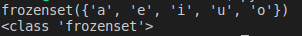# Sets in Python

## Sets in Python with Examples

In this article, I am going to discuss Sets in Python with examples. Please read our previous article where we discussed Tuples in Python with examples. As part of this article, we are going to discuss the following pointers which are related to Sets in Python.

1. What is the set data structure?
2. Creating a set
3. Difference between set() function and set class
4. Important function and methods of set:
5. Mathematical operations on the Set
6. Membership operators
7. Set Comprehension
8. Remove duplicates in list elements
9. Frozen set
10. Creating frozen set
11. Multiple Programs to understand above concepts
##### SET Data Structure in Python

Sets in python are also one of the data types just as lists and tuples. If we want to represent a group of unique elements then we can go for sets. Set cannot store duplicate elements.

##### Points to remember about set data structure in python:
1. Insertion order is not preserved.
2. Indexing and slicing are not allowed for the set.
3. Sets can store the same and different types of elements or objects.
4. Set objects are mutable which means once we create a set object we can perform any changes in it.
##### Creating a SET in Python:

We can create set by using curly braces {} and all elements separated by comma separator in set. Remember, [] is for lists, () is for tuples and {} for sets.

###### Example: Creating a set (demo1.py)
```s = {10,20,30,40}
print(s)
print(type(s))```

Output: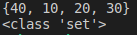###### Example: Creating a set with different elements (demo2.py)
```s = {10,'20','Rahul', 234.56, True}
print(s)
print(type(s))```
###### Output: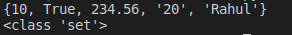###### Example: Creating a set using range function (demo3.py)
```s=set(range(5))
print(s)```

Output: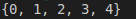From the above programs, we can observe that the order of the elements is not the same in the input and output. Hence, the order is not preserved.

###### Example: Duplicates not allowed (demo4.py)
```s = {10, 20, 30, 40, 10, 10}
print(s)
print(type(s))```
###### Output: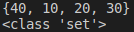##### Difference between set() function and set class in Python
1. set() is a predefined function in python.
2. The set() function is used to create a set of elements or objects, it takes a sequence as a parameter.
3. set is predefined class in python.
4. Once if we create a set then internally the created set will be represented as a set class type which can be checked by using the type function.
###### Example: Sets in Python Example (demo5.py)
```r=range(0, 10)
s=set(r)
print(s)
print(type(s))```
###### Output: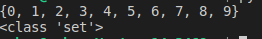##### Creating an empty set in Python:

We might think that creating an empty set is just to use empty curly braces {}. But, in python, we have another data type called dictionaries for which we use {} braces only. Hence creating a data type with empty curly braces will be considered as dictionary type rather than set. Then how to create an empty set? We can do so by using the set() function as shown in the below example.

###### Example: Creating an empty set (demo6.py)
```d={}
print(d)
print(type(d))

s=set()
print(s)
print(type(s))```

Output: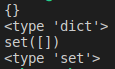#### IMPORTANT METHODS OF SETS in PYTHON:

The add() method in sets is used to add elements to the set

###### Example: add() function in sets (demo7.py)
```s={10,20,30}
print(s)```

Output: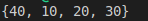##### update(x, y) method:

To add multiple items to the set. The arguments are not individual elements, but are Iterable objects like list, range etc. All elements present in the given Iterable objects will be added to the set.

###### Example: Update method in sets (demo8.py)
```s = {10,20,30}
l = [40,50,60,10]
s.update(l)
print(s)```

Output: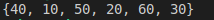###### Example: Update method (demo9.py)
```s = {10,20,30}
l = [40,50,60,10]
s.update(l, range(5))
print(s)```

Output: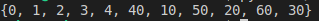##### Difference between add() and update() methods in set?
1. We can use add() to add individual items to the set, whereas we can use update() method to add multiple items to the set.
2. The add() method can take one argument whereas the update() method can take any number of arguments but the only point is all of them should be iterable objects.
###### Some examples
2. s.add(10,20,30) → Invalid, TypeError: add() takes exactly one argument (3 given)
3. s.update(10) → Invalid, TypeError: ‘int’ object is not iterable
4. s.update(range(1,10,2),range(0,10,2)) → Valid
##### copy() method in Set:

This method returns a copy of the set. It is a cloned object.

###### Example: copy() method (demo1o.py)
```s={10,20,30}
s1=s.copy()
print(s1)```

Output: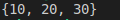##### pop() method:

This method removes and returns some random element from the set

###### Example: pop() method (demo11.py)
```s = {40,10,30,20}
print(s)
print(s.pop())
print(s)```
###### Output: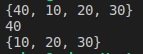##### remove(x) method in Set:

This method removes specific elements from the set. If the specified element is not present in the set then we will get KeyError.

###### Example: Remove() method (demo12.py)
```s={40,10,30,20}
s.remove(30)
print(s)```

Output: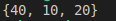###### Example: Remove (demo13.py)
```s={40,10,30,20}
s.remove(50)
print(s)```

Output: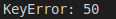This method removes the specified element from the set. If the specified element is not present in the set, then we won’t get any error.

```s={10,20,30}
print(s)```

Output: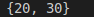```s={10,20,30}
print(s)
print(s)```

Output: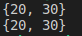##### clear() method in Python Sets:

This method removes all elements from the set.

###### Example: Clear() method(demo16.py)
```s={10,20,30}
print(s)
s.clear()
print(s)```

Output: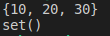##### union() method in set:

This method return all elements present in both sets.

Syntax: x.union(y) or x|y

###### Example: union() method (demo17.py)
```x={10,20,30,40}
y={30,40,50,60}
print(x.union(y))```

Output: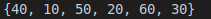##### intersection() method in Python SET:

This method returns common elements present in both x and y

Syntax: x.intersection(y) or x&y

###### Example: intersection() method(demo18.py)
```x = {10,20,30,40}
y = {30,40,50,60}
print(x.intersection(y))
print(x&y)```
###### Output: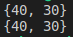##### difference() method in Python Sets:

This method returns the elements present in x but not in y

Syntax: x.difference(y) or x-y

###### Example: difference() method(demo19.py)
```x={10,20,30,40}
y={30,40,50,60}
print(x.difference(y))
print(x-y)
print(y-x)```
###### Output: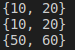##### symmetric_difference():

This method returns elements present in either x or y but not in both

Syntax: x.symmetric_difference(y) or x^y

###### Example: symmetric_difference() method(demo20.py)
```x={10,20,30,40}
y={30,40,50,60}
print(x.symmetric_difference(y))
print(x^y)```

Output: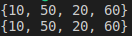##### MEMBERSHIP OPERATOR in SETS

The membership operators are, in and not in. By using these operators we can find whether the specified element exists in the set or not.

###### Example: membership operators in sets (demo21.py)
```s= {1, 2, 3, "Sharuk"}
print(s)
print(1 in s)
print('S' in s)
print(2 not in s)```
###### Output: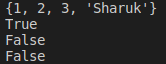##### SET COMPREHENSION in PYTHON

Just as we have seen list comprehensions, we also have set comprehensions.

###### Example: comprehensions in sets (demo22.py)
```s = {x*x for x in range(5)}
print(s)```

Output: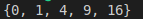##### REMOVING DUPLICATE ELEMENTS FROM SETS IN PYTHON

We can remove duplicates elements from a list by converting it to set using set() function

###### Example: remove duplicates elements in sets (demo23.py)
```l=[10,20,30,10,20,40]
s=set(l)
print(s)```

Output: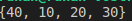##### FROZEN SET in PYTHON

As we know, a set is mutable which means we can modify the elements of a set after its creation. A frozen set is the same as a set but it is immutable which means we cannot modify the elements in the frozen set.

###### Creating a frozen set
```vowels = ('a', 'e', 'i', 'o', 'u')
fSet = frozenset(vowels)
print(fSet)
print(type(fSet))```
###### Output: